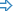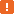# Searching for an ObjectQuite often, you may need to find an application’s object that matches certain criteria. This topic explains how you can do this.

### Searching for an Object in Keyword Tests

In keyword tests, you can search for objects using the Find Object operation. This operation searches for an object by its property values and then call a method or property of the found object. To configure this operation:

TestComplete will show the Operation Parameters wizard.

2. Specify the object TestComplete will search for and click Next.

3. Specify the list of object properties to check and the property values for the sought-for object:

• Enter the property name and value in the appropriate fields. These fields are case-insensitive.

• Repeat the previous steps to specify other properties that make up the search criteria.

• Click Next.

Notes:

• The specified information must uniquely identify the sought-for object, otherwise an incorrect object may be found.

• We do not recommend using the `VisibleOnScreen` property in search criteria. It can significantly decrease your test performance because it may take some time for TestComplete to get this property’s value, and TestComplete will have to get the value for every object it checks during the search.

4. Choose the found object's method to call and click Finish.

The operation result will be as follows:

• If the object with the specified property values was found, you get the result of the method call on the object.

• If the object was not found you will get a null object.

The Find Object operation captures only the first found object that matches the search criteria. To find all suitable objects, call the `FindAllChildren` method on the parent object. You can do this using the Call Object Method operation.

### Searching for an Object in Scripts

To search for an object from script tests, use the following methods:FindChildFindAllChildrenFind and FindAll

The methods use object properties as search criteria. They iterate through objects and check whether the object properties meet the specified criteria.We do not recommend using the `VisibleOnScreen` property in search criteria. It can significantly decrease your test performance because it may take some time for TestComplete to get this property’s value, and TestComplete will have to get the value for every object it checks during the search.
##### FindChild Method

The `FindChild` method has parameters to specify the properties and their values that the sought-for object must have and the maximum number of hierarchy level where the search is performed. The method’s result is either the found object or, if search failed, an empty “stub” object. To find out whether the search was successful, check the `Exists` property of the resulting object.

The following examples demonstrates how you can find an object by its caption. It searches for the Grad button in Windows Calculator, which is only available in the Calculator in the scientific mode (View > Scientific).

JavaScript, JScript

function Main()
{
var btn = Sys.Process("calc").FindChild("WndCaption", "Grad", 3);
if (btn.Exists)
btn.Click()
else
}

Python

``````def SearchByCaption():
if btn.Exists:
btn.Click()
else:

VBScript

Sub Main
Dim btn

Set btn = Sys.Process("calc").FindChild("WndCaption", "Grad", 3)
If btn.Exists Then
btn.Click
Else
End If
End Sub

DelphiScript

procedure Main;
var btn;
begin
if btn.Exists then
btn.Click
else
end;

C++Script, C#Script

function Main()
{
var btn = Sys["Process"]("calc")["FindChild"]("WndCaption", "Grad", 3);
if (btn["Exists"])
btn["Click"]()
else
}

The `FindChild` method also allows you to search for an object by multiple property values. To do this, call this method and specify arrays of property names and values, as shown in the example below. This example determines which number system is currently selected in Windows Calculator - Hex, Dec, Oct or Bin. For this purpose, the script searches for a radio button that is selected and has a three-letter caption. Note that the number system selection controls are available only in the Calculator’s scientific mode (View > Scientific).

JavaScript, JScript

function Main()
{
var calc = Sys.Process("calc");

var props = ["WndCaption", "wChecked"];
var values = ["???", true];

var btn = calc.FindChild(props, values, 3);
if (btn.Exists)
Log.Message("Current number system: " + btn.WndCaption);
else
Log.Error("Number system selection controls were not found. Most likely, the Calculator's Scientific view isn't active.");
}

Python

``````def SearchByMultyProps():
calc = Sys.Process("calc")

props = ["WndCaption", "wChecked"]
values = ["???", True]

btn = calc.FindChild(props, values, 3)
if (btn.Exists):
Log.Message("Current number system: " + btn.WndCaption);
else:
Log.Error("Number system selection controls were not found. Most likely, the Calculator's Scientific view isn't active.");``````

VBScript

Sub Main
Dim calc, props, values, btn

Set calc = Sys.Process("calc")

props = Array("WndCaption", "wChecked")
values = Array("???", True)

Set btn = calc.FindChild(props, values, 3)
If btn.Exists Then
Log.Message "Current number system: " & btn.WndCaption
Else
Log.Error "Number system selection controls were not found. Most likely, the Calculator's Scientific view isn't active."
End If
End Sub

DelphiScript

procedure Main;
var calc, props, values, btn;
begin
calc := Sys.Process('calc');

props := ['WndCaption', 'wChecked'];
values := ['???', true];

btn := calc.FindChild(props, values, 3);
if (btn.Exists) then
Log.Message('Current number system: ' + btn.WndCaption)
else
Log.Error('Number system selection controls were not found. Most likely, the Calculator''s Scientific view isn''t active.');
end;

C++Script, C#Script

function Main()
{
var calc = Sys["Process"]("calc");

var props = ["WndCaption", "wChecked"];
var values = ["???", true];

var btn = calc["FindChild"](props, values, 3);
if (btn["Exists"])
Log["Message"]("Current number system: " + btn["WndCaption"]);
else
Log["Error"]("Number system selection controls were not found. Most likely, the Calculator's Scientific view isn't active.");
}

##### FindAllChildren Method

To search for all objects that match certain criteria, use the `FindAllChildren` method applied to the parent object. This method is similar to `Find`, only it returns an array containing all found objects. To determine how many objects were found, check the length of the returned array.

The example below searches for all checked controls (radio buttons and check boxes) in the Windows Calculator’s window. Note, that the Calculator window contains these controls only in scientific mode (View > Scientific).

JavaScript, JScript

function Main()
{
var arr = Sys.Process("calc").FindAllChildren("wChecked", true, 4).toArray();
if (arr.length > 0)
{
for (var i = 0; i < arr.length; i++)
Log.Message(arr[i].WndCaption);
Log.Message("Total objects found: " + arr.length);
}
else
Log.Error("No objects were found.");
}

Python

``````def FindAllChildren():
arr = Sys.Process("calc").FindAllChildren("wChecked", True, 4)
if len(arr) > 0:
for i in range (0, len(arr)):
Log.Message(arr[i].WndCaption)
Log.Message("Total objects found: " + str(len(arr)))
else:
Log.Error("No objects were found.")``````

VBScript

Sub Main
Dim arr, i

arr = Sys.Process("calc").FindAllChildren("wChecked", True, 4)
If UBound(arr) >= 0 Then
For i = 0 To UBound(arr)
Log.Message arr(i).WndCaption
Next
Log.Message "Total objects found: " & (UBound(arr) + 1)
Else
Log.Error "No objects were found."
End If
End Sub

DelphiScript

procedure FindAllChildren;
var arr, n, i;
begin
arr := Sys.Process('calc').FindAllChildren('wChecked', true, 4);
n := VarArrayHighBound(arr, 1) + 1;
if n > 0 then
begin
for i := 0 to n - 1 do
Log.Message(arr[i].WndCaption);
Log.Message('Total objects found: ' + VarToStr(n));
end
else
Log.Error('No objects were found.');
end;

C++Script, C#Script

function Main()
{
var arr = Sys["Process"]("calc")["FindAllChildren"]("wChecked", true, 4)["toArray"]();
if (arr["length"] > 0)
{
for (var i = 0; i < arr["length"]; i++)
Log["Message"](arr[i]["WndCaption"]);
Log["Message"]("Total objects found: " + arr["length"]);
}
else
Log["Error"]("No objects were found.");
}

##### Find and FindAll Methods

There are also two additional search methods: `Find` and `FindAll`. They are analogous to `FindChild` and `FindAllChildren`, respectively, but also search in the parent object (the object to which the method is applied).

You can use the project property Object search strategy to choose the search order. You can change this option at run time via the `Options.Run.ObjectSearchStrategy` property. If the search is slow, try changing this option to the opposite of what it is set to. Note that the results may vary for different applications or even for different parts of the same application.